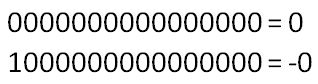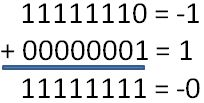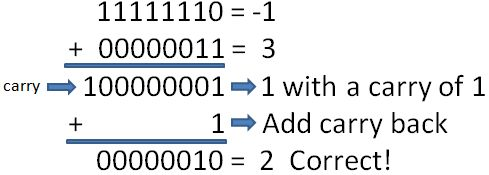# Back To Basics: IntegersI have been meaning for a while to start a beginners series of articles that will discuss a few basic concepts in software development. I had planned on starting at a level a bit above this one, but I had a comment on a recent post where I did something like this:

`  //multiply it by four and make sure it is positive`
`  return i > 0 ? i << 2 : ~(i << 2) + 1;`

This is very contrived (and that was the point in the post), but the part that got some people’s attention was “~(i << 2) + 1”. I’m assuming that because of the “+ 1” people thought that this was going to increment the value to one above what was returned by “i << 2”, but this is not the case.

The reason for this is the way in which the integers are represented in memory. Lets first start off by saying that there are two kinds of integers, signed and unsigned. Signed integers are integers that can represent both positive and negative numbers, while unsigned integers can only represent 0 and positive numbers. Most of you have probably heard of a “sign bit”, this is the leading bit in a signed binary number which tells us whether or not it is negative:A lot of people hear “sign bit” and think “Oh, well if that bit is 1 then this is a negative number, and if it is 0 then this is positive, but other than that the numbers are the same.” This statement is incorrect in most cases. Some people think it works like this:And it would be possible to implement a system like this, but no one does it. One problem being is that you have the issue of positive and negative zero:Now, of course there is no positive and negative zero, but in this system there are! And so, you would need special checks in the hardware to make sure that when comparing positive and negative zero that they would be equal. Another problem is that the signs have to be checked before a positive and negative number (or even two negative numbers) can be added:So, what just happened there? Well, binary arithmetic in this representation just doesn’t quite work. We have to come up with a bunch of special rules based on whether things are positive or negative. So, what do we do? Well, there is a second method called one’s complement.

In one’s complement a negative number is represented by the bitwise negation of the positive number:### Sidenote:

It is called a one’s complement because a bitwise NOT operation is equivalent to subtracting the binary number from an N-bit string of 1’s.

This system is better than the previous system, because you are now able to use standard binary addition to add any two numbers, but you still have the issue of positive and negative 0.Another issue that you can run into is that you have to keep track of the carry bit. So, say you added -1 and 3:Now, this may not sound too bad, but for performance reasons it is not optimal. So, now we come to two’s complement. This method makes a number negative by subtracting the positive representation from 2 to the power of N where N is the number of bits in the number. So, here is an example:So, you may be saying, “Hey, but now we have to perform a subtraction just to get a negative number.” Well, not exactly, because there is a shortcut for getting a two’s complement. You just apply a bitwise NOT and then add 1. Simple! Effective!Now you are probably wondering what is so special about this system, well, it fixes all our problems with the previous numbering systems! First, there is only one representation of zero. Secondly you can use normal binary addition for adding both positive and negative numbers. And third, you can ignore the carry bit, you don’t have to add it back in. So, if we add -1 and 3 in two’s complement, here is what happens:Now, how beautiful is that? And that is why virtually all computer systems in existence now use this system. It is by far the fastest and most efficient way of representing signed integers. Well, I hope that you enjoyed this refresher on integer number systems, I know that just going back through it was a great refresher for me! We often think that details like this aren’t important, and in most cases they aren’t, but isn’t knowing obscure implementation details what programming is all about?

Loved the article? Hated it? Didn’t even read it?

We’d love to hear from you.